Next: 11: TRUE AMPLITUDE MIGRATION Up: Goldin: Method of discontinuities Previous: Very simple but important

# 10: EXTREMAL VELOCITIES

We know how to determine the image of a reflector's location on a migrated section. This location, of course, depends on the choice of the continuation velocity vc. Being independent of a weight function, the location of caustics in the x,z-plane is determined by the system of equations(102)
and(103)

If at some velocity vc = vex the caustic and the image cross each other at a special point, we call this the extremal velocity vex. Usually we have a continuous interval of extremal velocities: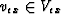.Typically for eachone can observe two or more special points. For some value vex = v*ex called the true extremal velocity, these special points unify in one point that appears as a bright spot.

Example: If the true model represents a homogeneous medium with velocity v and a planar reflector at depth h, the location of the reflector's image after CSP-migration is described by the system of equations (see Chapter 7):To find the location of caustics, we substitute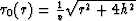and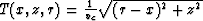.into system equations (102) and (103). So we have the system of equations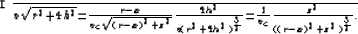If for someand r we haveand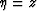, then the reflector's image contains the special point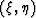.Calculations show that at vc < v there is no special point on the image. At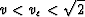we have two symmetrical special points which merge together into the one bright point when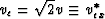(as shown in Figure).

Instead of a complete investigation of the situation, we can choose some particular ray of the eikonal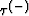.It is not difficult to determine the meaning offor the given velocity v=vc and the point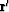belonging to the image and the ray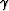; we can easily check condition (103). Let us again consider CSP-migration with the true model containing a curved reflector in a homogeneous medium (Figure, solid line). Let us choose the ray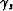which approaches the surface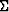vertically. This ray is connected with a stationary point rs of the function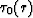:and we shall call this ray stationary. We shall denote h the true depth of the reflector and hm the image depth for the x=rs. If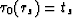, then hm satisfies(104)

It is easy to show that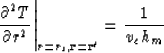(105)

The point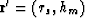belongs to a caustic if(106)
Using equation (104), we have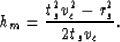(107)

Combining (106) and (107) we receive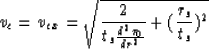(108)
It is easy to derive (see Figure)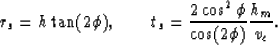(109)

As forwe can use standard techniques based on the continuation of wave front curvatures along the ray. We shall omit all these calculations and give the final result:(110)
where k is the reflector curvature at point. Substitution of equations (109) and (110) into (108) gives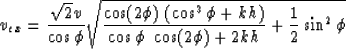(111)
If the reflector is planar (k=0), then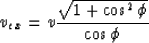(112)

If the pointis stationary for the reflector (), then(113)

If the reflector is planar and horizontal (k=0 and), then(114)
and, at last, if we have a point reflector (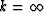), then

 vex = v (115)

Is this velocity vex the true extremal velocity? It is in all particular situations expressed by formulas (112) - (115).

In the zero offset case for arbitrary rays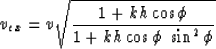and at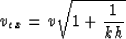If k=0 (planar reflector) we formally receive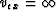, but in this case the amplitude does not actually depend on the meaning of the velocity vc.

In the common mid-point case travel-time curves for a point reflector and for a flat reflector are the same. It means that in this situation vex=v.Next: 11: TRUE AMPLITUDE MIGRATION Up: Goldin: Method of discontinuities Previous: Very simple but important
Stanford Exploration Project
1/13/1998